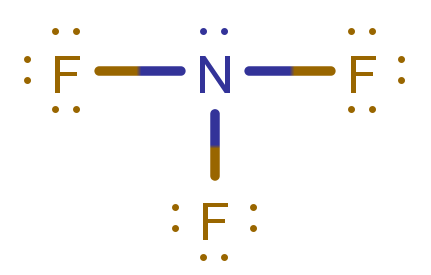# Problem: Write a hybridization and bonding scheme for each molecule. Label all bonds in NF3.

###### FREE Expert Solution

Step 1: Determine the central atom in this molecule.

• N less electronegative atom than F → central atom

Step 2: Calculate the total number of valence electrons present.

NF3:

Group               Valence Electrons

N         5A                   1 × 5 e = 5 e

F          7A                   3 × 7 e = 21 e

Total:  26 valence e

Step 3: Draw the Lewis Structure for the molecule

•  prefers to have 1 bond and complete octet
• surround N with three F atoms
• Distribute extra electrons in each of the F atoms (since they prefer to have complete octet)
• if there are remaining electrons, place it in the central atomStep 4: Determine the hybridization of the central atom.

• There are 4 domains or groups around central element N:
• 3 F atoms + 1 lone pair = 4 domains
• It will need 4 orbitals to accommodate these 4 groups.

Orbitals that can be used by the central atom:

Subshell                   Total # of orbitals

s           _                1 orbital

p       _ _ _              3 orbitals

d        _ _ _ _ _        5 orbitals

• 1 s orbital + 3 p orbitals = 4 orbitals (4 groups)
98% (215 ratings)###### Problem Details

Write a hybridization and bonding scheme for each molecule. Label all bonds in NF3.

Frequently Asked Questions

What scientific concept do you need to know in order to solve this problem?

Our tutors have indicated that to solve this problem you will need to apply the Hybridization concept. You can view video lessons to learn Hybridization. Or if you need more Hybridization practice, you can also practice Hybridization practice problems.